# CRÜCIAL PŸTHON Week 4: Numpy Broadcasting¶

In :
from IPython.core.display import Image
Image(url='http://labrosa.ee.columbia.edu/crucialpython/logo.png', width=600)

Out:In :
# Crucial imports
import numpy as np
import matplotlib.pyplot as plt

In :
# Let's say we have a (near) periodic signal
x = np.sin(np.arange(128))
plt.plot(x)

Out:
[<matplotlib.lines.Line2D at 0x10483afd0>]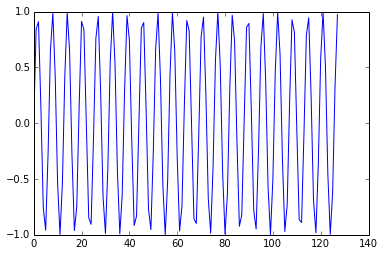To analyze time-varying content, we want to process individual overlapping frames.

We can use the stride_tricks from last week to get overlapped windows on a linear vector

In :
# Build a "framed" version of x as successive, overlapped sequences
# of frame_length points
from numpy.lib import stride_tricks
frame_length = 16
hop_length = 4
num_frames = 1 + (len(x) - frame_length) / hop_length
row_stride = x.itemsize * hop_length
col_stride = x.itemsize
x_framed = stride_tricks.as_strided(x,
shape=(num_frames, frame_length),
strides=(row_stride, col_stride))
plt.imshow(x_framed, interpolation='nearest', cmap='gray')

Out:
<matplotlib.image.AxesImage at 0x104907090>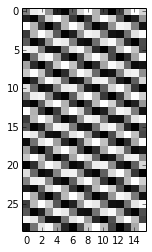In :
# If we take the FFT of each row, we can see the short-time fourier transform
plt.imshow(np.abs(np.fft.rfft(x_framed)), interpolation='nearest')

Out:
<matplotlib.image.AxesImage at 0x10493b8d0>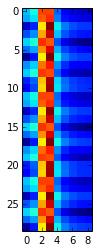In :
# Although there's a steady sinusoidal component, we see interference between the
# window frame and the signal phase.  We need a tapered window applied to each frame.
window = np.hanning(frame_length)
plt.plot(window)

Out:
[<matplotlib.lines.Line2D at 0x10496c4d0>]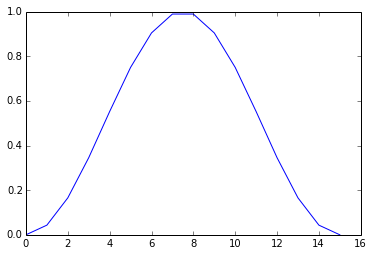In :
# But what's the best way to multiply each frame of x_framed by window?
# Linear algebra way is to multiply by a diagonal matrix
diag_window = np.diag(window)
plt.imshow(diag_window, interpolation='nearest', cmap='gray')

Out:
<matplotlib.image.AxesImage at 0x1049fae10>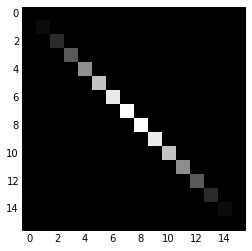In :
# Now apply it to each frame using matrix multiplication
x_framed_windowed = np.dot(x_framed, diag_window)
plt.imshow(x_framed_windowed, interpolation='nearest', cmap='gray')

Out:
<matplotlib.image.AxesImage at 0x104aa0190>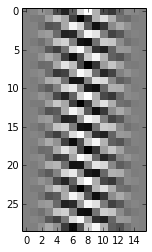In :
# Matlab way is to construct a matrix of repeating rows of the same size
window_repeated = np.tile(window, (num_frames,1))
plt.imshow(window_repeated, interpolation='nearest', cmap='gray')
# then pointwise multiplication applies it to each row
x_framed_windowed = x_framed * window_repeated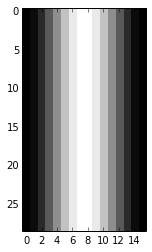In :
# Numpy broadcasting implicitly (and efficiently) repeats singleton or missing dimensions
# to make matrices the same size, so tiling is unneeded
plt.imshow(x_framed*window, interpolation='nearest', cmap='gray')

Out:
<matplotlib.image.AxesImage at 0x104b2c6d0>In :
# Compare the timings:
%timeit np.dot(x_framed, np.diag(window))
%timeit x_framed*np.tile(window, (num_frames,1))
%timeit x_framed*window
# The big win is not having to allocate the second num_frames x frame_length array

100000 loops, best of 3: 10.7 µs per loop
100000 loops, best of 3: 15 µs per loop
100000 loops, best of 3: 4.13 µs per loop

In :
# What about if we had our data in columns?
x_framed_T = x_framed.T
plt.imshow(x_framed_T * window, interpolation='nearest', cmap='gray')

---------------------------------------------------------------------------
ValueError                                Traceback (most recent call last)
<ipython-input-12-33858fc02e56> in <module>()
2 x_framed_T = x_framed.T
----> 3 plt.imshow(x_framed_T * window, interpolation='nearest', cmap='gray')

ValueError: operands could not be broadcast together with shapes (16,29) (16) 
In :
# Broadcast works by starting at the last dimensions (the fastest-changing ones in 'C' ordering)
# and promoting either one if it's one.
# So we just have to make our window be frame_length x 1
# We can do this with slicing and np.newaxis:
plt.imshow(x_framed_T * window[:,np.newaxis], interpolation='nearest', cmap='gray')

Out:
<matplotlib.image.AxesImage at 0x104b0b7d0>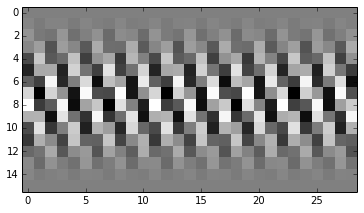In :
# Broadcasting works across multiple dimensions.
# It goes through each dimension from the last, looking for a match
# or promoting singletons
a = np.random.rand( 3, 4, 5 )
b = np.random.rand( 3, 5 )
c = a*b

---------------------------------------------------------------------------
ValueError                                Traceback (most recent call last)
<ipython-input-14-a039b1ac09b5> in <module>()
4 a = np.random.rand( 3, 4, 5 )
5 b = np.random.rand( 3, 5 )
----> 6 c = a*b

ValueError: operands could not be broadcast together with shapes (3,4,5) (3,5) 
In :
# That didn't work because there was no singleton dimension to promote
# so we can introduce one, with reshape for instance:
b2 = np.reshape(b, (3, 1, 5))
c1 = a*b2
# or using slicing
c2 = a * b[:, np.newaxis, :]
print np.allclose(c1, c2)

True


For the full description of how broadcasting works, see the SciPy documentation: http://docs.scipy.org/doc/numpy/user/basics.broadcasting.html

In :
# Remember why we wanted the windowing, to remove artefacts from the STFT?
plt.subplot(121)
plt.imshow(np.abs(np.fft.rfft(x_framed)), interpolation='nearest')
plt.subplot(122)
plt.imshow(np.abs(np.fft.rfft(x_framed * window)), interpolation='nearest')
# Windowing drastically reduces framing-related artefacts
# (at the cost of a little frequency resolution)

Out:
<matplotlib.image.AxesImage at 0x104c354d0>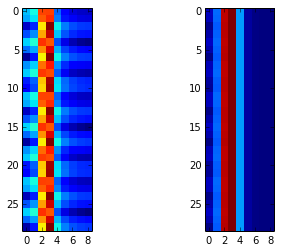In [ ]: